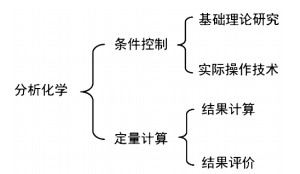## Modularization of the Knowledge of Analytical Chemistry According to the Function,, , ,Abstract

This paper will integrate the theoretical teaching with the laboratory teaching by modular constructing of the knowledge content. Because the core task of quantitative analytical chemistry is the analysis of quantity, it is divided into condition controlling and quantitative calculation. The condition control is the premise for the quantitative analysis. This part is divided into theoretical study and practical techniques. The ultimate goal of the quantitative analysis is to obtain the results. The quantitative calculation is divided into two parts, results calculation and evaluation. After modularization according to the function, the knowledge structure of analytical chemistry is made clear, and has vertical hierarchical and horizontal comparability, which will foster students' ability to make a summary of knowledge, analyze and solve problems. In such a way, students can not only grasp knowledge content, but also improve autonomous learning ability, and finally realize learning for life.

Keywords： Analytical chemistry ; Modularization ; Condition controlling ; Quantitative calculation

TAO Ran, ZHAO Wenxiu, DONG Shuguo, BAI Yu. Modularization of the Knowledge of Analytical Chemistry According to the Function. University Chemistry[J], 2018, 33(6): 60-64 doi:10.3866/PKU.DXHX201801009

## 1 教学中的问题

### 图1#### 2.1.1 基础理论研究

 滴定反应类型 化学平衡 滴定曲线 指示剂 过程 计量点 突跃范围 影响因素 变色原理 变色点及范围 酸碱反应 ${{K}_{\text{a}}}$ 四点 $\text{pH}=-\frac{1}{2}(\lg \frac{{{K}_{\text{W}}}\cdot {{K}_{\text{a}}}}{{{c}_{\text{sp}}}})$ ±0.1% c、${{K}_{\text{a}}}$ 酸式 $\text{p}{{\text{H}}_{\text{HIn}}}$ 碱式 $\text{p}{{\text{H}}_{\text{HIn}}}\pm 1$ 配位反应 $K_{\text{MY}}^{'}$ 四点 $\text{p}{{\text{M}}^{'}}=\frac{1}{2}(\lg {{c}_{\text{M}(\text{SP})}}+\lg K_{\text{MY}}^{'})$ ±0.1% c、$K_{\text{MY}}^{'}$ 自身配合物 $\text{p}{{\text{M}}_{\text{t}}}=\lg {{K}_{\text{MIn}}}-\lg {{\alpha }_{\text{In}(\text{H})}}$ 氧化还原反应 ${{K}^{'}}$ 四点 ${{\varphi }_{\text{sp}}}=\frac{{{n}_{1}}\varphi _{1}^{'}+{{n}_{2}}\varphi _{2}^{'}}{{{n}_{1}}+{{n}_{2}}}$ ±0.1% $\Delta {{\varphi }^{'}}$ 氧化态 $\varphi _{\text{InOx/InRed}}^{'}$ 还原态 $\varphi _{\text{InOx/InRed}}^{'}\pm 1$ 沉淀反应 ${{K}_{\text{sp}}}$ 四点 $\text{pCl}=-\frac{1}{2}\lg {{K}_{\text{sp}}}$ ±0.1% c、${{K}_{\text{sp}}}$ 游离态被吸附 被吸附

#### 2.1.2 实际操作条件

 滴定类型 滴定方法 标准溶液 试样预处理 滴定操作 配制 基准物质 酸度 温度 酸碱滴定 间接法 无水碳酸钠 不调节 常温 常规 配位滴定 间接法 氧化锌 缓冲溶液 常温 常规 氧化还原滴定 碘量法 间接法 重铬酸钾 中性或弱酸性 常温 初始，快滴慢摇；近终点时，慢滴快摇 高锰酸钾法 草酸钠 硫酸 70–80 ℃ 慢快慢 亚硝酸钠法 对氨基苯磺酸 盐酸 < 15/15–25 ℃ 快速滴定法 沉淀滴定 铬酸钾法 间接法 氯化钠 中性或弱碱性 常温 剧烈振摇 铁铵矾法 硝酸 剧烈振摇 吸附指示剂法 利于显色 常规

##### 2.2.1 结果计算

 滴定类型 化学反应方程式 典型示例 酸碱滴定 $m{{\text{H}}_{n}}\text{A}+n\text{B(OH}{{\text{)}}_{m}}\to (m\times n){{\text{H}}_{\text{2}}}\text{O}+{{\text{B}}_{n}}{{\text{A}}_{m}}$ 混合碱的测定 配位滴定 $\text{M}+\text{Y}\to \text{MY}$ 水中总硬度的测定 氧化还原滴定 $a\text{O}{{\text{x}}_{1}}+b\text{Re}{{\text{d}}_{2}}\to c\text{Re}{{\text{d}}_{1}}+d\text{O}{{\text{x}}_{2}}$ 过氧化氢含量的测定 沉淀滴定 $\text{A}{{\text{g}}^{+}}+{{\text{X}}^{-}}\to \text{AgX}$ 生理盐水中氯化钠含量的测定

##### 2.2.2 结果评价

 阶段 内容 目的 公式 1 求统计量 求平均值、标准偏差等 $\overline{x}=\frac{1}{n}\sum\limits_{i=1}^{n}{{{x}_{i}}}; S=\sqrt{\frac{\sum\limits_{i=1}^{n}{d_{i}^{2}}}{n-1}}=\sqrt{\frac{\sum\limits_{i=1}^{n}{{{\left( {{x}_{i}}-\overline{x} \right)}^{2}}}}{n-1}}$ 2 可疑值取舍 看是否有异常值 ${{Q}_{计}}=\frac{\left| {{x}_{疑}}-{{x}_{邻}} \right|}{{{x}_{最大}}-{{x}_{最小}}}$或${{G}_{计}}=\frac{\left| {{x}_{异常}}-\overline{x} \right|}{S}$ 3 F检验 看是否有显著的偶然误差，评价数据的精密度 $F=\frac{S_{1}^{2}}{S_{2}^{2}}\left( {{S}_{1}}>{{S}_{2}} \right)$ 4 t检验 看是否有系统误差，评价数据的准确度 ${{t}_{计}}\text{=}\left| \overline{x}-\mu \right|\cdot \frac{\sqrt{n}}{S}$或${{t}_{计}}\text{=}\frac{\left| \overline{{{x}_{1}}}-\overline{{{x}_{2}}} \right|}{{{S}_{\text{R}}}}\cdot \sqrt{\frac{{{n}_{1}}\cdot {{n}_{2}}}{{{n}_{1}}+{{n}_{2}}}}$

## 参考文献 原文顺序 文献年度倒序 文中引用次数倒序 被引期刊影响因子

Kellner R. ; Mermet I. M. ; Otto M. Analytical Chemistry 1st ed Weinheim: Wiley-VCH, 1998.

/

 〈〉